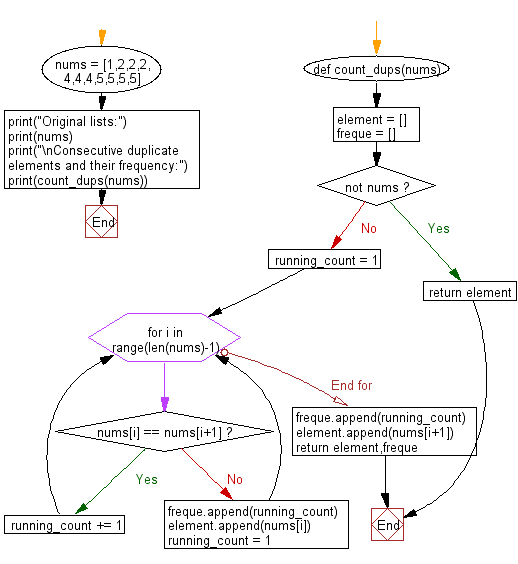﻿ Python: Count the frequency of consecutive duplicate elements in a given list of numbers - w3resource# Python: Count the frequency of consecutive duplicate elements in a given list of numbers

## Python List: Exercise - 131 with Solution

Write a Python program to count the frequency of consecutive duplicate elements in a given list of numbers.

Sample Solution:

Python Code:

``````def count_dups(nums):
element = []
freque = []
if not nums:
return element
running_count = 1
for i in range(len(nums)-1):
if nums[i] == nums[i+1]:
running_count += 1
else:
freque.append(running_count)
element.append(nums[i])
running_count = 1
freque.append(running_count)
element.append(nums[i+1])
return element,freque

nums = [1,2,2,2,4,4,4,5,5,5,5]
print("Original lists:")
print(nums)

print("\nConsecutive duplicate elements and their frequency:")
print(count_dups(nums))
```
```

Sample Output:

```Original lists:
[1, 2, 2, 2, 4, 4, 4, 5, 5, 5, 5]

Consecutive duplicate elements and their frequency:
([1, 2, 4, 5], [1, 3, 3, 4])
```

Flowchart:## Visualize Python code execution:

The following tool visualize what the computer is doing step-by-step as it executes the said program:

Python Code Editor:

Have another way to solve this solution? Contribute your code (and comments) through Disqus.

What is the difficulty level of this exercise?

Test your Python skills with w3resource's quiz

﻿

## Python: Tips of the Day

```print(2_000_000)
```2000000